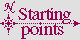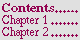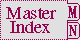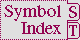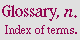#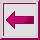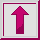Function APPLY

Syntax:

apply function &rest args+ => result*

Arguments and Values:

function---a function designator.

results---the values returned by function.

Description:

Applies the function to the args.

When the function receives its arguments via &rest, it is permissible (but not required) for the implementation to bind the rest parameter to an object that shares structure with the last argument to apply. Because a function can neither detect whether it was called via apply nor whether (if so) the last argument to apply was a constant, conforming programs must neither rely on the list structure of a rest list to be freshly consed, nor modify that list structure.

setf can be used with apply in certain circumstances; see Section 5.1.2.5 (APPLY Forms as Places).

Examples:

``` (setq f '+) =>  +
(apply f '(1 2)) =>  3
(setq f #'-) =>  #<FUNCTION ->
(apply f '(1 2)) =>  -1
(apply #'max 3 5 '(2 7 3)) =>  7
(apply 'cons '((+ 2 3) 4)) =>  ((+ 2 3) . 4)
(apply #'+ '()) =>  0

(defparameter *some-list* '(a b c))
(defun strange-test (&rest x) (eq x *some-list*))
(apply #'strange-test *some-list*) =>  implementation-dependent

(defun bad-boy (&rest x) (rplacd x 'y))
(bad-boy 'a 'b 'c) has undefined consequences.
(apply #'bad-boy *some-list*) has undefined consequences.
```

``` (defun foo (size &rest keys &key double &allow-other-keys)
(let ((v (apply #'make-array size :allow-other-keys t keys)))
(if double (concatenate (type-of v) v v) v)))
(foo 4 :initial-contents '(a b c d) :double t)
=>  #(A B C D A B C D)
```

Affected By: None.

Exceptional Situations: None.